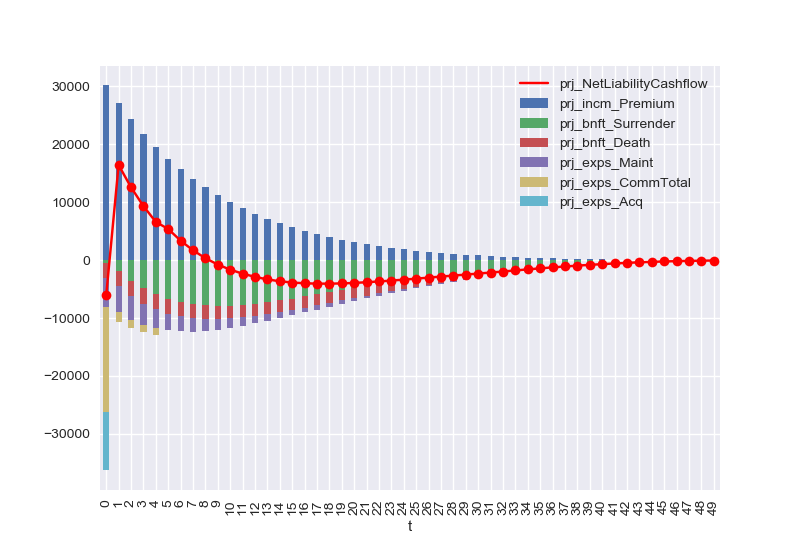# simplelife cashflow¶

Draw a graph of liability cashflows of a simple whole life policyOut:

Started loading data from 'input.xlsm'.
Input space and its sub spaces are saved in 'lifelib.mx'.
You can load input data from the saved file instead of 'input.xlsx'
by passing 'load_saved=True' to simplelife.build function.


try:
import simplelife.simplelife as simplelife
except ImportError:
import simplelife

proj = simplelife.build().Projection

'prj_bnft_Surrender',
'prj_bnft_Death',
'prj_exps_Maint',
'prj_exps_CommTotal',
'prj_exps_Acq']

polid = 171

for cells in vars:
list(proj[polid].cells[cells](t) for t in range(50))

cfs = proj[polid].frame[vars].sort_index().dropna()
cfs[vars[1:]] = cfs[vars[1:]].mul(-1)

[proj[polid].prj_NetLiabilityCashflow[t] for t in range(50)]

ncf = proj[polid].prj_NetLiabilityCashflow.frame.sort_index()

import seaborn as sns
sns.set()

axes = ncf.plot.line(marker='o', color='r')
cfs.plot(kind='bar', stacked=True, ax=axes)


Total running time of the script: ( 0 minutes 22.469 seconds)

Gallery generated by Sphinx-Gallery